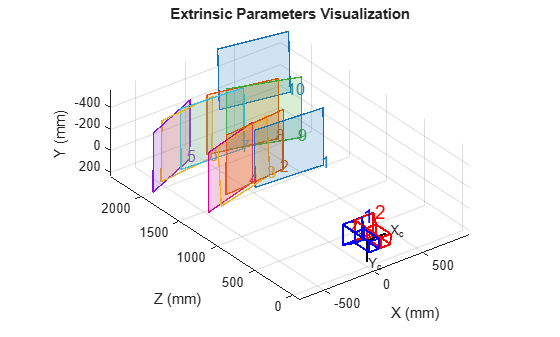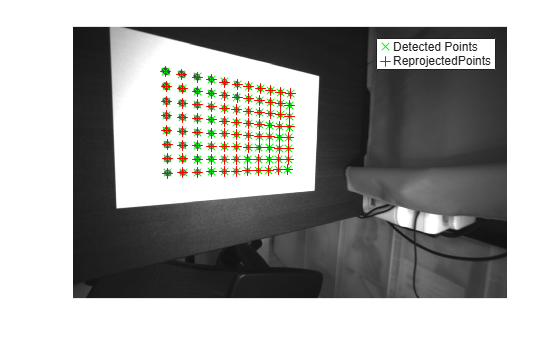# estimateCameraParameters

Calibrate a single or stereo camera

## Syntax

``````[cameraParams,imagesUsed,estimationErrors] = estimateCameraParameters(imagePoints,worldPoints)``````
``````[stereoParams,pairsUsed,estimationErrors] = estimateCameraParameters(imagePoints,worldPoints)``````
``cameraParams = estimateCameraParameters(___,Name,Value)``

## Description

example

``````[cameraParams,imagesUsed,estimationErrors] = estimateCameraParameters(imagePoints,worldPoints)``` returns `cameraParams`, a `cameraParameters` object containing estimates for the intrinsic and extrinsic parameters and the distortion coefficients of a single camera. The function also returns the images you used to estimate the camera parameters and the standard estimation errors for the single camera calibration. The `estimateCameraParameters` function estimates extrinsics and intrinsics parameters.```

example

``````[stereoParams,pairsUsed,estimationErrors] = estimateCameraParameters(imagePoints,worldPoints)``` returns `stereoParams`, a `stereoParameters` object containing the parameters of the stereo camera. The function also returns the images you used to estimate the stereo parameters and the standard estimation errors for the stereo camera calibration.```

example

````cameraParams = estimateCameraParameters(___,Name,Value)` configures the `cameraParams` object properties specified by one or more `Name,Value` pair arguments, using any of the preceding syntaxes. Unspecified properties have their default values.```

## Examples

collapse all

Create a set of calibration images.

```images = imageSet(fullfile(toolboxdir('vision'),'visiondata',... 'calibration','mono')); imageFileNames = images.ImageLocation;```

Detect the calibration pattern.

`[imagePoints, boardSize] = detectCheckerboardPoints(imageFileNames);`

Generate the world coordinates of the corners of the squares.

```squareSizeInMM = 29; worldPoints = generateCheckerboardPoints(boardSize,squareSizeInMM);```

Calibrate the camera.

```I = readimage(images,1); imageSize = [size(I, 1),size(I, 2)]; params = estimateCameraParameters(imagePoints,worldPoints, ... 'ImageSize',imageSize);```

Visualize the calibration accuracy.

`showReprojectionErrors(params);`Visualize camera extrinsics.

```figure; showExtrinsics(params);````drawnow;`

Plot detected and reprojected points.

```figure; imshow(imageFileNames{1}); hold on; plot(imagePoints(:,1,1), imagePoints(:,2,1),'go'); plot(params.ReprojectedPoints(:,1,1),params.ReprojectedPoints(:,2,1),'r+'); legend('Detected Points','ReprojectedPoints'); hold off;```Specify calibration images.

```leftImages = imageDatastore(fullfile(toolboxdir('vision'),'visiondata', ... 'calibration','stereo','left')); rightImages = imageDatastore(fullfile(toolboxdir('vision'),'visiondata', ... 'calibration','stereo','right'));```

Detect the checkerboards.

```[imagePoints,boardSize] = ... detectCheckerboardPoints(leftImages.Files,rightImages.Files);```

Specify the world coordinates of the checkerboard keypoints. Square size is in millimeters.

```squareSize = 108; worldPoints = generateCheckerboardPoints(boardSize,squareSize);```

Calibrate the stereo camera system. Both cameras have the same resolution.

```I = readimage(leftImages,1); imageSize = [size(I,1),size(I,2)]; params = estimateCameraParameters(imagePoints,worldPoints, ... 'ImageSize',imageSize);```

Visualize the calibration accuracy.

` showReprojectionErrors(params);`Visualize camera extrinsics.

```figure; showExtrinsics(params);```Create a set of calibration images.

```imds = imageDatastore(fullfile(toolboxdir('vision'),'visiondata',... 'calibration','circleGrid','mono')); calibrationImages = readall(imds); calibrationImages = cat(4,calibrationImages{:});```

Define the circle grid pattern dimensions.

`patternDims = [8 11];`

Detect the pattern in the calibration images.

```imagePoints = detectCircleGridPoints(calibrationImages, patternDims,... 'PatternType','symmetric');```

Specify the world coordinates for the circle grid keypoints. Center distance is in millimeters.

```centerDistance = 18; worldPoints = generateCircleGridPoints(patternDims,centerDistance,... 'PatternType','symmetric');```

Calibrate the camera using the calibration images.

```imageSize = size(calibrationImages,1:2); params = estimateCameraParameters(imagePoints,worldPoints,... 'ImageSize',imageSize);```

Plot the detected pattern grid and the reprojected points.

```figure imshow(calibrationImages(:,:,:,1)) hold on plot(imagePoints(:,1,1), imagePoints(:,2,1),'gx','MarkerSize',8) plot(params.ReprojectedPoints(:,1,1),params.ReprojectedPoints(:,2,1),'r+','MarkerSize',8) legend('Detected Points','ReprojectedPoints') hold off```## Input Arguments

collapse all

Key points of calibration pattern, specified as an array of [x,y] intrinsic image coordinates.

CalibrationInput Array of [x,y] Key Points
Single Camera

M-by-2-by-numImages array of [x,y] points.

• The number of images, numImages, must be greater than or equal to 2.

• The number of keypoint coordinates in each pattern, M, must be greater than 3.

Partially detected patterns are only supported for single camera calibration. To include partially detected patterns in the estimate, use [`NaN,NaN`] as x-y coordinates for missing keypoints.

Stereo Camera

M-by-2-by-numPairs-by-2 array of [x,y] points.

• numPairs is the number of stereo image pairs containing the calibration pattern.

• The number of keypoint coordinates in each pattern, M, must be greater than 3.

• `imagePoints`(`:,:,:,1`) are the points from camera 1.

• `imagePoints`(`:,:,:,2`) are the points from camera 2.

Data Types: `single` | `double`

Key points of calibration pattern in world coordinates, specified as an M-by-2 array of M number of [x,y] world coordinates. The pattern must be planar; therefore, z-coordinates are zero.

Data Types: `single` | `double`

### Name-Value Arguments

Specify optional pairs of arguments as `Name1=Value1,...,NameN=ValueN`, where `Name` is the argument name and `Value` is the corresponding value. Name-value arguments must appear after other arguments, but the order of the pairs does not matter.

Before R2021a, use commas to separate each name and value, and enclose `Name` in quotes.

Example: `'WorldUnits'`,`'mm'` sets the world point units to millimeters.

World points units, specified as the comma-separated pair consisting of `'WorldUnits'` and a character vector or string scalar.

Estimate skew, specified as the comma-separated pair consisting of `'EstimateSkew'` and a logical scalar. When you set this property to `true`, the function estimates the image axes skew. When set to `false`, the image axes are exactly perpendicular and the function sets the skew to zero.

Number of radial distortion coefficients to estimate, specified as the comma-separated pair consisting of `'NumRadialDistortionCoefficients'` and the value `2` or `3`.

Radial distortion is the displacement of image points along radial lines extending from the principal point.

• As image points move away from the principal point (positive radial displacement), image magnification decreases and a pincushion-shaped distortion occurs on the image.

• As image points move toward the principal point (negative radial displacement), image magnification increases and a barrel-shaped distortion occurs on the image.The radial distortion coefficients model this type of distortion. The distorted points are denoted as (xdistorted, ydistorted):

xdistorted = x(1 + k1*r2 + k2*r4 + k3*r6)

ydistorted= y(1 + k1*r2 + k2*r4 + k3*r6)

• x, y — Undistorted pixel locations. x and y are in normalized image coordinates. Normalized image coordinates are calculated from pixel coordinates by translating to the optical center and dividing by the focal length in pixels. Thus, x and y are dimensionless.

• k1, k2, and k3 — Radial distortion coefficients of the lens.

• r2 = x2 + y2

Typically, two coefficients are sufficient for calibration. For severe distortion, such as in wide-angle lenses, you can select three coefficients to include k3.

Tangential distortion flag, specified as the comma-separated pair consisting of, `'EstimateTangentialDistortion'` and a logical scalar. When you set this property to `true`, the function estimates the tangential distortion. When you set it to `false`, the tangential distortion is negligible.

Tangential distortion occurs when the lens and the image plane are not parallel. The tangential distortion coefficients model this type of distortion.The distorted points are denoted as (xdistorted, ydistorted):

xdistorted = x + [2 * p1 * x * y + p2 * (r2 + 2 * x2)]

ydistorted = y + [p1 * (r2 + 2 *y2) + 2 * p2 * x * y]

• x, y — Undistorted pixel locations. x and y are in normalized image coordinates. Normalized image coordinates are calculated from pixel coordinates by translating to the optical center and dividing by the focal length in pixels. Thus, x and y are dimensionless.

• p1 and p2 — Tangential distortion coefficients of the lens.

• r2 = x2 + y2

Initial guess for camera intrinsics, specified as the comma-separated pair consisting of `'InitialIntrinsicMatrix'` and a 3-by-3 matrix. If you do not provide an initial value, the function computes the initial intrinsic matrix using linear least squares.

Initial guess for radial distortion coefficients, specified as the comma-separated pair consisting of `'InitialRadialDistortion'` and a 2- or 3-element vector. If you do not provide an initial value, the function uses `0` as the initial value for all the coefficients.

Image size produced by camera, specified as the comma-separated pair consisting of '`ImageSize`' and as 1-by-2 [mrows, ncols] vector.

## Output Arguments

collapse all

Camera parameters, returned as a `cameraParameters` object.

Images you use to estimate camera parameters, returned as a P-by-1 logical array. P corresponds to the number of images. The array indicates which images you used to estimate the camera parameters. A logical `true` value in the array indicates which images you used to estimate the camera parameters.

The function computes a homography between the world points and the points detected in each image. If the homography computation fails for an image, the function issues a warning. The points for that image are not used for estimating the camera parameters. The function also sets the corresponding element of `imagesUsed` to `false`.

Standard errors of estimated parameters, returned as a `cameraCalibrationErrors` object or a `stereoCalibrationErrors` object.

Camera parameters for stereo system, returned as a `stereoParameters` object. The object contains the intrinsic, extrinsic, and lens distortion parameters of the stereo camera system.

Image pairs used to estimate camera parameters, returned as a P-by-1 logical array. P corresponds to the number of image pairs. A logical `true` value in the array indicates which image pairs you used to estimate the camera parameters.

## Algorithms

collapse all

### Calibration Algorithm

You can use the Camera Calibrator app with cameras up to a field of view (FOV) of 95 degrees.

The calibration algorithm assumes a pinhole camera model:

`$w\left[\begin{array}{ccc}x& y& 1\end{array}\right]=\left[\begin{array}{cccc}X& Y& Z& 1\end{array}\right]\left[\begin{array}{c}R\\ t\end{array}\right]K$`

 (X,Y,Z): world coordinates of a point (x,y): coordinates of the corresponding image point w: arbitrary scale factor K: camera intrinsic matrix R: matrix representing the 3-D rotation of the camera t: translation of the camera relative to the world coordinate system

Camera calibration estimates the values of the intrinsic parameters, the extrinsic parameters, and the distortion coefficients. There are two steps involved in camera calibration:

1. Solve for the intrinsics and extrinsics in closed form, assuming that lens distortion is zero. 

2. Estimate all parameters simultaneously including the distortion coefficients using nonlinear least-squares minimization (Levenberg–Marquardt algorithm). Use the closed form solution from the preceding step as the initial estimate of the intrinsics and extrinsics. Then set the initial estimate of the distortion coefficients to zero. 

 Zhang, Z. “A Flexible New Technique for Camera Calibration”. IEEE Transactions on Pattern Analysis and Machine Intelligence.Vol. 22, No. 11, 2000, pp. 1330–1334.

 Heikkila, J, and O. Silven. “A Four-step Camera Calibration Procedure with Implicit Image Correction”, IEEE International Conference on Computer Vision and Pattern Recognition, 1997.

 Bouguet, J.Y. “Camera Calibration Toolbox for Matlab”, Computational Vision at the California Institute of Technology.

 Bradski, G., and A. Kaehler. Learning OpenCV : Computer Vision with the OpenCV Library. Sebastopol, CA: O'Reilly, 2008.